• 开放寻址法

2019-09-22 16:13:49
开放寻址开放寻址法插入关键字查找关键字开放寻址法探查序列的计算方法 开放寻址法   解决哈希表(在一些文献中又称作散列表)冲突的方法有：链接法(chaining) 和 开放寻址法(open addressing)。本文讲解开放寻址...

开放寻址法的装载因子

给定一个能存放n个元素的、具有m个槽位的哈希表T，采用开放寻址法时T的装载因子为： α = n / m ， n ≤ m \alpha=n/m，n \leq m

开放寻址法

在开放寻址法中，所有元素都存在哈希表里。也就是说，每个表项或包含动态集合的一个元素，或包含 N I L NIL (空)。当查找某个元素时，要系统地检查所有的表项，直到找到所需的元素或者最终查明该元素不在表中。在开放寻址法中，哈希表可能被填满，以至于不能插入任何新的元素，该方法导致的一个结果便是装载因子 α \alpha 绝对不会超过1。
开放寻址法的好处是其不使用指针，直接使用
哈希函数
来算出存取的位置，这样也可以加快存取速度。

插入关键字

为了使用开放寻址法插入一个元素，需要连续地检查哈希表，或称为探查(probe)，直到找到一个空槽来放置待插入的关键字为止。检查的顺序依赖待插入的关键字。对于每一个关键字 k k ，使用开放寻址法探查序列为 < h ( k , 0 ) , h ( k , 1 ) , ⋯   , h ( k , m − 1 ) > <h(k,0), h(k,1),\cdots, h(k,m-1)> ，假设散列表T中的元素仅为关键字，关键字 k k 等同于包含关键字 k k 的元素。 T T 中的每一个槽或包含一个关键字，或包含 N I L NIL (如果该槽为空)。 H A S H − I N S E R T HASH-INSERT 过程以一个哈希表 T T 和一个关键字 k k 为输入，要么返回其关键字 k k 的存储槽位，要么因为散列表已满而返回出错标志。

HASH-INSERT(T, k)
i=0
repeat
j=h(k,i)
if T[j] == NIL
T[j] = k
return j
else i=i+1
until i==m
error “hash table overflow"

查找关键字

查找关键字k的算法的探查序列与将k插入时的算法一样。因此，在查找的过程中碰到一个空槽时(该位置为NIL)，查找算法就停止，因为如果 k k 在表中，它就应该在此处，而不会在探查序列随后的位置上。过程HASH-SEARCH的输入为一个哈希表 T T 和一个关键字 k k ，如果槽 j j 中包含了关键字 k k ，则返回 j j ；如果 k k 不存在 T T 中，则返回 N I L NIL

HASH-SEARCH(T, k)
i=0
repeat
j=h(k,i)
if T[j] == NIL
T[j] = k
return j
else i=i+1
until i==m
return NIL

删除关键字

从开放寻址法的哈希表中删除元素比较麻烦。当我们从槽 i i 中删除关键字时，不能仅仅将其置为 N I L NIL 来标识其为空。如果这样做，就会有问题：在插入关键字 k k 时，发现槽 i i 被占用了，则 k k 就被插入到后面的位置上；如果此时将槽 i i 中的关键字删除后，就无法检索到关键字 k k 了。有个解决办法，就是在槽 i i 中置一个特殊的值 D E L E T E D DELETED 替代 N I L NIL 来标记该槽。这样就要对过程 H A S H − I N S E R T HASH-INSERT 做相应的修改，将这样的槽当做空槽，是得在此仍然可以插入新的关键字。对 H A S H − S E A R C H HASH-SEARCH 无需做改动，因为它在搜索时会绕过 D E L E T E D DELETED 标识。但是查找时间就不依赖于装载因子了。
这里给出的算法参考了Knuth 6.4 Algorithm R(TAOCP)，该算法对应使用线性探测来确定关键字 k k 插入位置的HASH-INSERT过程的关键字k删除算法。 H A S H − L I N E A R − P R O B E − D E L E T E HASH-LINEAR-PROBE-DELETE 过程以一个哈希表 T T 和一个关键字 k k 为输入。该过程没有使用删除标记 D E L E T E D DELETED 这种方式，而是将关键字 k k 删除后，将该位置之后的关键字做移动这种方式(即重新调整各个关键字的顺序)。
$(i+1) %m$

nextIndex(i,m)
return (i + 1 <= m + 1) ? (i+1) : 1

使用线性探测确定关键字 k k 的索引

HASH-LINEAR-PROBE(T, k)
for i=0 to m-1
index = (h(k) + i) mod m
if T[index] == k or T[index] == NIL
return index
return NIL

HASH-LINEAR-PROBE-DELETE(T, k)
i = HASH-SEARCH(T,k)
if T[i] == NIL
return
T[i] = NIL
j = i
for i = i+1 to nextIndex(i,m)
if T[i] == NIL
return
r = HASH-LINEAR-PROBE(T, T[i])
if r == NIL //无法探测到T[i]应该存放的位置
return
if i <= r < j or r<j<i or j<i<=r  //r循环地位于i和j之间
continue
if r != NIL and r != i //T[i]存放的位置不应该是当前位置
T[j] = T[i]
T[i] = NIL
j = i
i = i+1

开放寻址法探查序列的计算方法

有三种技术常用来计算开放寻址法中的探测序列：线性探查、二次探查和双重探查。这几种技术都能保证对每个关键字 k , < h ( k , 0 ) , h ( k , 1 ) , ⋯   , h ( k , m − 1 ) > k,<h(k,0), h(k,1),\cdots, h(k,m-1)> 都是 < 0 , 1 , ⋯   , m − 1 > <0, 1,\cdots, m-1> 的一个排列。但是，这些技术都不能满足均匀哈希的假设，因为它们能产生的不同探查序列数都不超过 m 2 m^2 个(均匀散列要求有 m ! m! 个探查序列)。在三种技术中，双重探查产生的探查序列最多，似乎能给出最好的效果。

1. 线性探查(linear probing)
给定一个普通的哈希函数 h ′ : U → 0 , 1 , ⋯   , m − 1 h':U\to{0,1,\cdots,m-1} ，称之为辅助哈希函数，线性探查方法采用的哈希函数为：
h ( k , i ) = ( h ′ ( k ) + i )    m o d    m ,    i = 0 , 1 , ⋯   , m − 1 h(k,i) = (h'(k) + i) \; mod \;m,\; i=0,1,\cdots,m-1
给定一个关键字 k k ，首先探查 T [ h ′ ( k ) ] T[h'(k)] （此时的 i = 0 i=0 ），即由辅助哈希函数所给出的槽位。再探查 T [ h ′ ( k ) + 1 ] T[h'(k)+1] ，以此类推，直至槽 T [ m − 1 ] T[m-1] 。然后又绕到槽 T [ 0 ] T T [ 1 ] T ⋯ \cdots ，直到最后探查到槽 T [ h ′ ( k ) − 1 ] T[h'(k)-1] 。在线性探查方法中，初始探查位置绝对了整个序列，故只有 m m 种不同的探查序列。
线性探查法比较容易实现，但它存在着一个问题，称为一次群集。随着连续被占用的槽不断增加，平均查找时间也随之不断增加。群集现象很容易出现，这是因为当一个空槽前有 i i 个满的槽时，该空槽为下一个将被占用的概率是 ( i + 1 ) / m (i+1)/m 。连续被占用的槽就会变得越来越长因而平均查找时间也会越来越大。
2. 二次探查
二次探查采用如下形式的哈希函数：
h ( k , i ) = ( h ′ ( k ) + c 1 i + c 2 i 2 )    m o d    m ,    i = 0 , 1 , ⋯   , m − 1 h(k,i) = (h'(k) + c_1i + c_2i^2) \; mod \;m,\; i=0,1,\cdots,m-1
其中 h ′ h' 是一个辅助哈希函数， c 1 c_1 c 2 c_2 为正的辅助常数， i = 0 , 1 , ⋯   , m − 1 i=0,1,\cdots,m-1 。初始的探查位置为 T [ h ′ ( k ) ] T[h'(k)] ，后续的探查位置要加上一个偏移量，该偏移量是一个关于 i i 的二次函数。这种探查方法的效果比线性探查好得多，但是，为了能够充分利用哈希表， c 1 c_1 c 2 c_2 m m 的值要受到限制。此外，如果两个关键字的初始探查位置相同，那么它们的探查序列也是相同的，这是因为 h ( k 1 , 0 ) = h ( k 2 , 0 ) h(k_1,0)=h(k_2,0) 蕴含着 h ( k 1 , i ) = h ( k 2 , i ) h(k_1,i)=h(k_2,i) 。像线性探查一样，初始探测位置决定了整个序列，这样也仅有 m m 个不同的探查序列。
3. 双重哈希
双重哈希是用于开放寻址法的最好方法之一，因为它所产生的排列具有随机选择排列的许多特性。双重哈希采用如下的哈希函数：
h ( k , i ) = ( h 1 ( k ) + i h 2 ( k ) )    m o d    m ,    i = 0 , 1 , ⋯   , m − 1 h(k,i) = (h_1(k) + ih_2(k)) \; mod \;m,\; i=0,1,\cdots,m-1
其中 h 1 h_1 h 2 h_2 均为辅助哈希函数。初始探查位置为 T [ h 1 ( k ) ] T[h_1(k)] ，后续的探查位置加上偏移量 h 2 h_2 。因此，不像线程探查或者二次探查，这里的探查序列以两种不同方式依赖于关键字 k k ，因为初始探查位置、偏移量或者二者都可能发生变化。
为了能查找整个哈希表，值 h 2 ( k ) h_2(k) 必须要与表的大小 m m 互素(习题11.4-4)。有一种简便的方法确保这个条件成立，就是 m m 为2的幂，并设计一个总产生奇数的 h 2 h_2 。另一种方法是取m为素数，并设计一个总是返回较 m m 小的正整数的函数 h 2 h_2
一个结论：如果对某个关键字 k k m m h 2 ( k ) h_2(k) 有最大公约数 d ≥ 1 d\geq1 ，则在对关键字 k k 的一次不成功查找中，在返回槽 h 1 ( k ) h_1(k) 之前，要检查散列表中 1 / d 1/d 个元素。于是，当 d = 1 d=1 时， m m h 2 ( k ) h_2(k) 互素，查找操作可能要检查整个哈希表。
展开全文• 开放寻址法中，所有的元素都存放在散列表里。也就是说，每个表项或包含动态集合的一个元素，或包含NoneNoneNone。当查找某个元素时，要系统地检查所有的表项，直到找到所需的元素，或者最终查明该元素不在表中。不...

在开放寻址法中，所有的元素都存放在散列表里。也就是说，每个表项或包含动态集合的一个元素，或包含 N o n e None 。当查找某个元素时，要系统地检查所有的表项，直到找到所需的元素，或者最终查明该元素不在表中。不像链接法，这里既没有链表，也没有元素存放在散列表外。因此在开放寻址法中，散列表可能会被填满，以至于不能插入任何新的元素。该方法导致的一个结果便是装载因子 a a 绝对不会超过1。

开放寻址法的核心思想是，如果出现了散列冲突，我们就重新探测一一个空闲位置，将其插入。比如，我们可以使用线性探测法。当我们往散列表中插入数据时，如果某个数据经过散列函数散列之后，存储位置已经被占用了，我们就从当前位置开始，依次往后查找，看是否有空闲位置，如果遍历到尾部都没有找到空闲的位置，那么我们就再从表头开始找，直到找到为止。

当然，也可以将用作链接的链表存放在散列表未用的槽中，但开放寻址法的好处就在于它不用指针，而是计算出要存取的槽序列。于是，不用存储指针而节省的空间，使得可以用同样的空间来提供更多的槽，潜在地减少了冲突，提高了检索速度。

为了使用开放寻址法插入一个元素，需要连续地检查散列表，或称为探查，直到找到一个空槽来放置待插入的关键字为止。检查的顺序不一定是 0 , 1 , ⋯   , m − 1 0, 1, \cdots, m-1 ，而是要依赖于待插入的关键字。为了确定要探查哪些槽，我们将散列函数加以扩充，使之包含探查号以作为其第二个输入参数。这样，散列函数就变为：
h : U × { 0 , 1 , ⋯   , m − 1 } → { 0 , 1 , ⋯   , m − 1 } h:U×\{0, 1, \cdots, m-1\}\rightarrow \{0, 1, \cdots, m-1\}
对每一个关键字 k k ，使用开放寻址法的探查序列是 < 0 , 1 , ⋯   , m − 1 > <0, 1, \cdots, m-1> 的一个排列，使得当散列表逐渐填满时，每一个表位最终都可以被考虑为用来插入新关键字的槽。

查找关键字 k k 的算法的探查序列与将 k k 插入时的算法一样。因此，查找过程中碰到一个空槽时，查找算法就（非成功地）停止，因为如果 k k 在表中，它就应该在此处，而不会在探查序列随后的位置上（之所以这样说，是假定了关键字不会从散列表中删除）。

从开放寻址法的散列表中删除操作元素比较困难。当我们从槽 i i 中删除关键字时，不能仅将 N o n e None 置于其中来标识它为空。如果这样做，就会有问题：在插入关键字 k k 时，发现槽 i i 被占用了，则就被插人到后面的位置上；此时将槽 i i 中的关键字删除后，就无法检索到关键字 k k 了。

有一个解决办法，就是在槽 i i 中置一个特定的值 D E L E T E D DELETED 替代 N o n e None 来标记该槽。这样就将这样的一个槽当做空槽，使得在此仍然可以插入新的关键字。

线性探查

给定一个普通的散列函数 h ′ : U → { 0 , 1 , ⋯   , m − 1 } h':U\rightarrow \{0, 1, \cdots, m-1\} ，称之为辅助，线性探查方法采用的散列函数为：
h ( k , i ) = ( h ′ ( k ) + i ) m o d    m h(k ,i)=(h'(k)+i)\mod m\quad
给定一个关键字 k k ，首先探查槽 T [ h ′ ( k ) ] T[h'(k)] ，即由辅助散列函数所给出的槽位。再探查槽T T [ h ′ ( k ) + 1 ] T[h'(k)+1] ，依此类推，直至槽 T [ m − 1 ] T[m-1] 。然后，又绕到槽 T [ 0 ] , T [ 1 ] , ⋯ T, T, \cdots 直到最后探查到槽 T [ h ′ ( k ) − 1 ] T[h'(k)-1] 。在线性探查方法中，初始探查位置决定了整个序列，故只有 m m 种不同的探查序列。

线性探査方法比较容易实现，但它存在着一个问题，称为一次群集。随着连续被占用的槽不断增加，平均査找时间也随之不断增加。群集现象很容易出现，这是因为当个空槽前有 i i 个满的槽时，该空槽为下一个将被占用的概率是 i + 1 m \frac{i+1}{m} 。连续被占用的槽就会变得越来越长，因而平均查找时间也会越来越大。

二次探查

二次探查采用如下形式的散列函数：
h ( k , i ) = ( h ′ ( k ) + c 1 i + c 2 i 2 ) m o d    m h(k ,i)=(h'(k)+c_1i+c_2i^2)\mod m\quad
其中 h ′ h' 是一个辅助散列函数， c 1 c_1 c 2 c_2 为正的辅助常数。初始的探查位置为 T [ h ′ ( k ) ] T[h'(k)] ，后续的探查位置要加上一个偏移量，该偏移量以二次的方式依赖于探查序号 i i 。这种探查方法的效果要比线性探查好得多，但是，为了能够充分利用散列表， c 1 c_1 c 2 c_2 m m 的值要受到限制。此外，如果两个关键字的初始探查位置相同，那么它们的探查序列也是相同的，这是因为 h ( k 1 , 0 ) = h ( k 2 , 0 ) h(k_1,0)=h(k_2,0) 蕴涵着 h ( k 1 , i ) = h ( k 2 , i ) h(k_1,i)=h(k_2,i) 。这一性质可导致一种轻度的群集，称为二次群集。像在线性探查中一样，初始探查位置决定了整个序列，这样也仅有 m m 个不同的探查序列被用到。

双重散列

双重散列是用于开放寻址法的最好方法之一，因为它所产生的排列具有随机选择排列的许多特性。双重散列采用如下形式的散列函数：
h ( k , i ) = ( h 1 ( k ) + i h 2 ( k ) ) m o d    m h(k ,i)=(h_1(k)+ih_2(k))\mod m\quad

其中 h 1 h_1 h 2 h_2 均为辅助散列函数。初始探查位置为 T [ h 1 ( k ) ] T[h_1(k)] ，后续的探查位置是前一个位置加上偏移量 h 2 ( k ) h_2(k) m m 。因此，不像线性探查或二次探查，这里的探查序列以两种不同方式依赖于关键字 k k ，因为初始探查位置、偏移量或者二者都可能发生变化。下图给出了一个使用双重散列法进行插入的例子。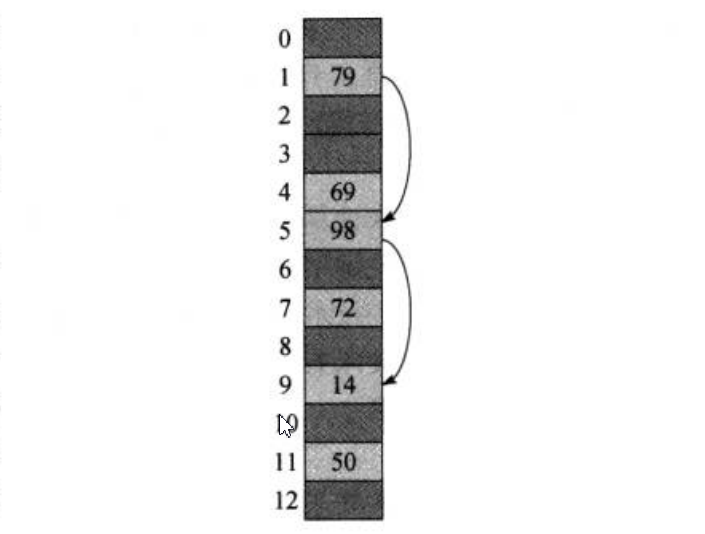为了能查找整个散列表，值 h 2 ( k ) h_2(k) 必须要与表的大小 m m 互质。当 m m 为素数或者2的幂时，双重散列法中用到了 Θ ( m 2 ) \Theta(m^2) 种探查序列，而线性探查或二次探查中用了 Θ ( m ) \Theta(m) 种，故前者是后两种方法的种改进。因为每一对可能的 ( h 1 ( k ) , h 2 ( k ) (h_1(k), h_2(k) 都会产生一个不同的探查序列。因此，对于 m m 的每一种可能取值，双重散列的性能看起来就非常接近“理想的”均匀散列的性能。

尽管除素数和2的幂以外的 m m 值在理论上也能用于双重散列中，但是在实际中，要高效地产生 h 2 ( k ) h_2(k) 确保使其与 m m 互质，将变得更加困难。部分原因是这些数的相对密度中可能较小。

开放寻址散列的分析

像在链接法中的分析一样，开放寻址法的分析也是以散列表的装载因子 a = n m a=\frac{n}{m} 来表达的当然，使用开放寻址法，每个槽中至多只有一个元素，因而 n ≤ m n≤m ，也就意味着 a ≤ 1 a≤1

假设采用的是均匀散列。在这种理想的方法中，用于插入或查找每一个关键字 k k 的探查序列等可能地为 < 0 , 1 , ⋯   , m − 1 > <0, 1, \cdots, m-1> 的任意一种排列。当然，每一个给定的关键字有其相应的唯一固定的探查序列。我们这里想说的是，考虑到关键字空间上的概率分布及散列函数施于这些关键字上的操作，每一种探查序列都是等可能的。

现在就来分析在均匀散列的假设下，用开放寻址法来进行散列时探查的期望次数。则：

1. 给定一个装载因子为 a = n m < 1 a=\frac{n}{m}<1 的开放寻址散列表，并假设是均匀散列的，则对于一次不成功的查找，其期望的探查次数至多为 1 1 − a \frac{1}{1-a}
2. 假设采用的是均匀散列，平均情况下，向一个装载因子为 a a 的开放寻址散列表中插入一个元素至多需要做 1 1 − a \frac{1}{1-a} 次探查。
3. 对于一个装载因子为 a < 1 a<1 的开放寻址散列表，一次成功查找中的探查期望数至多为 1 a ln ⁡ 1 1 − a \frac{1}{a}\ln\frac{1}{1-a}
展开全文算法 算法导论 数据结构 哈希表 散列表
• 开放寻址——线性探测 分离链接散列算法还有一个亟待解决的缺点：需要指针，由于给新单元分配地址需要时间，这就导致了速度减慢，所以不太好。还有，因为链表是次第关联的结构，实现算法的代码自身的复杂程度和出错...

开放寻址——线性探测

https://www.cnblogs.com/hongshijie/p/9419387.html
分离链接散列算法还有一个亟待解决的缺点：需要指针，由于给新单元分配地址需要时间，这就导致了速度减慢，所以不太好。还有，因为链表是次第关联的结构，实现算法的代码自身的复杂程度和出错概率会大大增加。而只要采用这种策略，就很难保证每组冲突的词条在空间上能够彼此毗邻，因为动态分配的节点在内存里不一定是连续的，这样一来会导致一个致命缺陷：对于稍大规模的词条集合，查找中将做大量的I／O操作，无法利用系统预先缓存，导致效率低下。

因此或许我们应该放弃这种策略，并反其道而行之，仅仅依靠基本的散列表结构，就地排解冲突反而是更好的选择。也就是采用所谓的开放定址策略，它的特点在于：散列表所占用的空间在物理上始终是地址连续的一块，相应的所有的冲突都在这块连续空间中加以排解。而无需向分离链接那样申请额外的空间。对！所有的散列以及冲突排解都在这样一块封闭的空间内完成。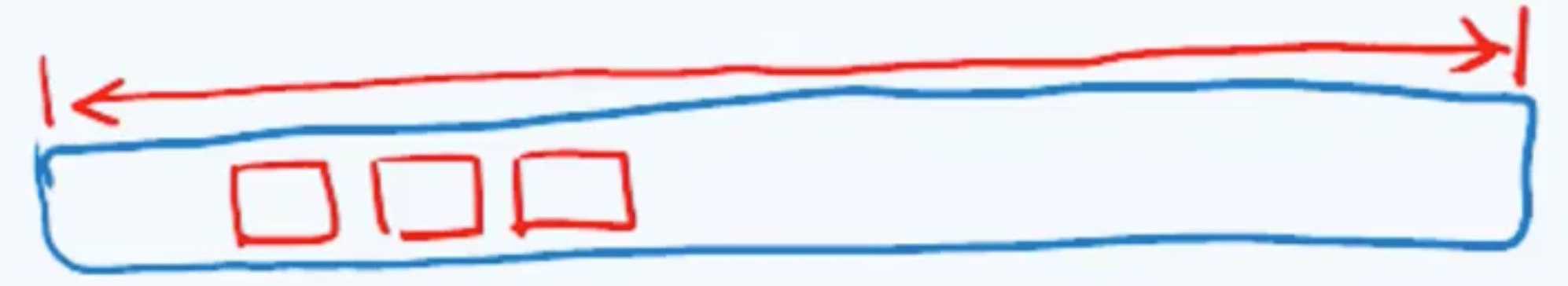因此相应地，这种策略也可以称作为闭散列。如果有冲突发生，就要尝试选择另外的单元，直到找到一个可供存放的空单元。具体存放在哪个单元，是有不同优先级的，优先级最高的是他原本归属的那个单元。从这个单元往后，都按照某种优先级规则排成一个序列，而在查找的时候也是按着这个序列行进，每个词条对应的这个序列被称为探测序列or查找链。

抽象来说，就是我们遇到冲突后，会相继尝试h0(x),h1(x),h2(x)这些单元，其中hi(x)= ( Hash( x ) + F ( I ) ) % TableSize，并且约定F(0)=0，F（x）是解决冲突的方法，就是刚才说的那个“优先级规则”。因为所有的数据都要放在这块空间，所以开放定址所需要的表规模比分离链接要大。通常而言开放定址法的装填因子lambda应该低于0.5。而根据对不同F(x)的选择，学界划分出三种常用的探测序列：线性探测法、平方探测法、双散列

1.线性探测法

在线性探测法中，函数F是关于i的线性函数，典型的情形是F(i)=i。这相当于逐个探测每个单元（必要时可以绕回）以查找出一个空单元。下面显示了将{89,18,49,58,69}插入到一个散列表中的情况（竖着看），使用了和之前一样的散列函数hash(x)=x%size，他们有冲突怎么办？用F(i)=i这个方法，每次从i=0开始尝试，那么根据hi(x)= ( Hash( x ) + F ( I ) ) % TableSize就可以计算出各自不相冲突的地址了。完美！（暂时的）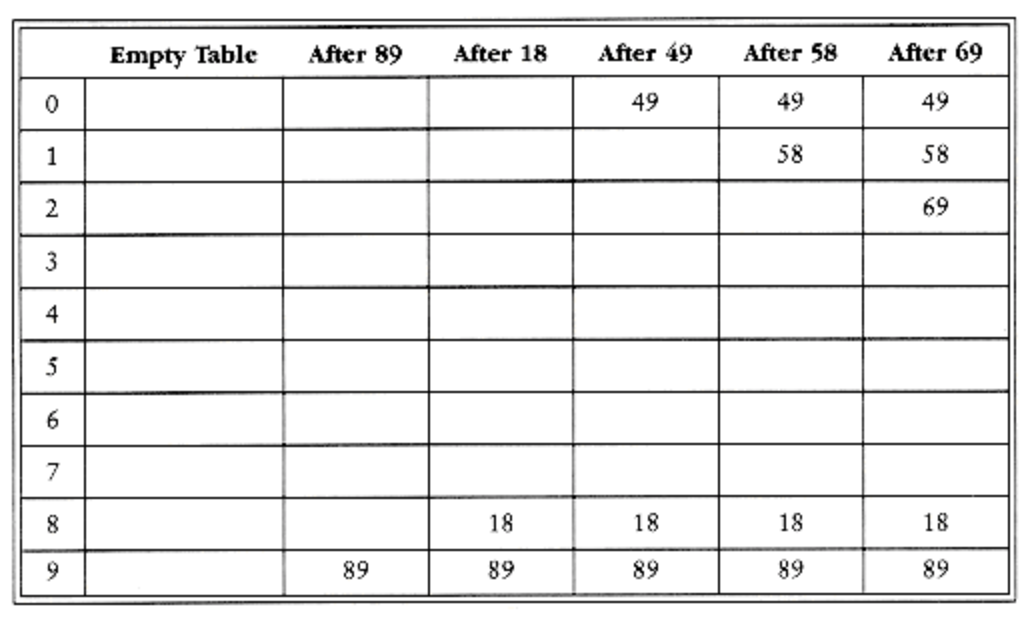我们脑内单步调试一下：第一个冲突在49产生:（49%10+0）%10=9，被89占了，那接着往后试，i=1，（49%10+1）%10=0，空的，放入这个空闲地址，这个地址是开放的。58依次和18,89,49产生冲突，试选三次后才找到一个空单元。对69的冲突也如此解决，一旦冲突，试探紧邻其后的单元，直至找到空单元or抵达散列表末尾。线性探测序列0->1->2->3在物理上保持连贯性的，具有局部性，这样一来系统的缓存作用将得到充分发挥，而对于大规模的数据集，这样一来更是可以减少I/O的次数。只要表足够大，总能找到一个空闲单元，但是这太费时间了。更糟的是——就算一开始空闲区域多，经过多次排解冲突后，数据所占据的单元也会开始形成一些区块，聚集在一起，被称为一次聚集(primary clustering)，但在前面动机篇里说过，散列函数的初衷是避免数据扎堆，所以后面必须改进。

那么总体看来散列到区块的任何关键字都需要多次试选单元才能解决冲突，然后被放到对应的那个区块里。下面做一个总结

优点：

无需附加空间（指针、链表、溢出区）
探测序列具有局部性，可以利用系统缓存，减少IO
缺点：

耗费时间>O(1)
冲突增多——以往的冲突会导致后续的连环冲突，发生惨烈的车祸光看这个图可能没什么感觉，举个例子吧，这样感触更深。我们开一个size=7的散列表，也保证了size是素数。把{0，1，2，3，7}，就按这个顺序依次插入。前四个数都没问题，依次插入没有冲突。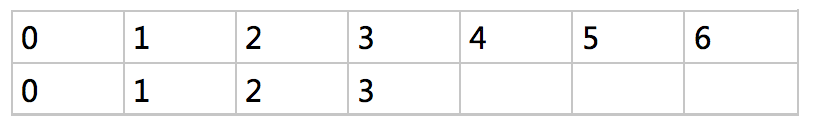但是为了插入7，我们先试探0发现非空，往后走，依次试探1,2,3都非空，直到4可以放进去。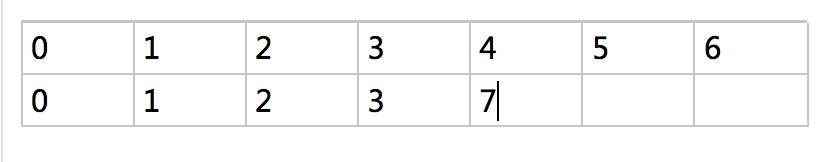在这个散列表的生存期里只有1个发生冲突。看似很棒对吧，再来看另一插入次序：{7，0，1，2，3}。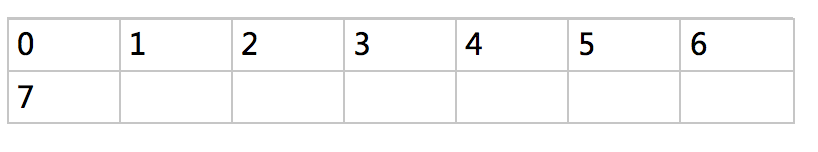插入7没问题，但插入0的时候就有冲突了，实际上自此之后每一个数插入都会遇到冲突，前后对比可以看出，第二种插入顺序发生的很多冲突本来是可以避免的。这个时候想必我们改进这种策略的意愿就十分迫切了。要支持词条的删除则需要格外的小心，现在我们来做一探讨。按照线性探测的规则，先后插入彼此冲突的一组词条都将存放在同一个查找序列中，而更确切的讲：它们应该按照逻辑次序构成整个查找链的一个前缀，其中不得有任何的空桶缝隙。因此词条的删除操作需要做额外的一些处理，如果我们不做一些事先准备，直接将词条删除（就类似对于链表，删除节点的时候不做链条调整，而直接free那个单元，那不直接凉了），就会造成查找链断裂，后续词条丢失——明明存在但访问不到。

对于这种连续空间的单元删除，一个直观的构想是：将后续词条悉数取出，再重新插入。但这太特么慢了，时间复杂度爆炸。其实对于这个问题有一种典型的处理手法：lazy delete，仅做一个删除标记，比如里面预留一个del变量，设置为TRUE。那么在日后查找中，遇到他之后就应该越过继续往后查找而不是在此返回。在插入时遇到，就把它视作一个空单元，数据覆盖即可。应该说针对开放定址策略，懒惰删除不仅是“不得已而为之”的方法，甚至可以说是针对这种情况的最优方法。因为毕竟在开放定址策略中，每一个桶单元都同时属于多个查找链

开放寻址散列的分析
定理11.6 ：
一个装载因子a=n/m<1的开放寻址散列表，在一次不成功的查找中，期望的探查数之多为1/(1-a).假设散列是均匀的。
推论11.7
平均情况，向一个装载因子为a的开放寻址散列表中插入一个元素时，至多需要做1/(1-a)次探查。假设采用的是均匀散列。
定理11.8
给定一个装载因子为a<1的开放寻址散列表，一次成功查找中的期望探查数之多为(1/a)ln(1/(1-a)).

#include "iostream"
#include "math.h"
#define Null -1
#define DELETED -2
using namespace std;

//散列函数（全域散列在“全域散列解决哈希冲突中已讲”）
int Hash_div(int val, int m)//除法求余。val值，m为槽的个数(是一个不为2的幂的质数)
{
return val % m;
}

int Hash_multi(int val, int m)//乘法散列。m一般为2的某次幂
{
double A = (sqrt(5) - 1) / 2;
return ((val*A - int(val*A)) * m);
}

//开放寻址方法：线性探查、二次探查、双重探查
int Line_Prob(int val, int m, int i) //m为槽的个数(是一个不为2的幂的质数),m越大查找次数就越少
{
return (Hash_div(val, m) + i) % m;
}

int Twice_Prob(int val, int m,int i)
{
int c1 = 1, c2 = 3;
return (Hash_div(val, m) + c1 * i + c2 * i*i) % m; //其中常数c1,c2,m的值的选择是受限制的
}
int Double_Probe(int val, int m, int i)
{
return (Hash_div(val, m) + i * (1 + Hash_div(val, m-1))) % m; //其中m为质数，还可以选择m为2的幂,并设置一个总产生奇数的hash_div2
}
int hash_insert1(int T[], int m, int k)
{
int i = 0;
do
{
int j = Line_Prob(k, m, i);//这里可以替换成二次，双重探查。插入，查找，删除函数同时被替换
if (T[j] == Null || T[j] == DELETED)
{
T[j] = k;
return j;
}
else i++;
} while (i != m);
cerr << "hash table overflow" << endl;
}
int hash_search(int T[], int m, int k)
{
int i = 0, j = 0;
do
{
j = Line_Prob(k, m, i);//这里可以替换成二次，双重探查。插入，查找，删除函数同时被替换
if (T[j] == k)
{
return j;
}
else i++;
} while (T[j] != Null || i != m);
return Null;
}
void hash_delete(int T[], int m, int k)
{
int j = hash_search(T, m, k);//首先先找到该关键字k
if (j != Null)
{
T[j] = DELETED;//如果找到了，那么设置其为空。
cout << "删除成功！" << endl;
}
else cout << "待删除的数据不在表中！" << endl;
}
void Display(int T[],int m)
{

cout << "散列表信息显示:"  ;
for (int i = 0; i < m; i++)
{
cout << T[i] << " ";
}
cout << endl;
}
void main()
{
int a = { 10,22,31,4,15,28,17,88,59 };
int T = { 0 };
for (int i = 0; i<19; i++)
{
T[i] = Null;
}
for (int i = 0; i<9; i++)
{
hash_insert1(T, 19, a[i]);
}
Display(T,19);
hash_delete(T, 19, 10);
Display(T, 19);
system("pause");
}

展开全文• #include<iostream> #include<cstring> using namespace std; const int N=200003; int h[N],null=0x3f3f3f3f; int find(int x) { int k=(x%N+N)%N; while(h[k]!=null&&...}...

#include<iostream>
#include<cstring>
using namespace std;
const int N=200003;
int h[N],null=0x3f3f3f3f;
int find(int x)
{
int k=(x%N+N)%N;
while(h[k]!=null&&h[k]!=x)
{
k++;
if(k==N) k=0;
}
return k;
}
int main()
{
int n;
scanf("%d",&n);
memset(h,0x3f,sizeof h);
while(n--)
{
char op;
int x;
scanf("%s%d",op,&x);
int k=find(x);
if(*op='I') h[k]=x;
else
{
if(h[k]!=null) puts("Yes");
else puts("No");
}
}
return 0;

展开全文c++ 算法
• 开放寻址法和拉链法十分类似，只是处理冲突的方式不一样。拉链法通过在冲突位置开链表解决，开放寻址法通过往后顺次找空位置解决。 拉链法： #include<iostream> #include<cstdio> #include<vector&...链表 数据结构
• hash table(开放寻址法-线性探查实现的哈希表） // // Created by 许加权 on 2021/7/17. // #ifndef C11LEARN_HASHLINER_H #define C11LEARN_HASHLINER_H #include "KeyNode.h" template<typename T> class ...数据结构 算法导论 hashjava 数据结构
• 开放寻址法   3.再HASH法   4.建立公共溢出池   等等 为什么不用3和4？ 4方法浪费内存，3增加了算法的复杂度，不推荐。其实我也不清楚。 链式地址法和开放地址法的优缺点分别是什么？ 链式地址法（HashMap...
• 开放寻址法：又称开放定址法，当哈希冲突发生时，从发生冲突的那个单元起，按照一定的次序，从哈希表中寻找一个空闲的单元，然后把发生冲突的元素存入到该单元。这个空闲单元又称为开放单元或者空白单元。开放寻址法...列表 数据结构 python java 哈希表
• 开放寻址法VS链表法

千次阅读 2019-07-05 09:21:04
开放寻址法 只用数组一种数据结构存储，继承了数组的优点，对CPU缓冲友好，易于序列化。但是对内存的利⽤率并不如链表法，且冲突的代价更高。当数据量⽐较⼩、装载因⼦⼩的时候，适合采⽤开放寻址法。这也是Java中的...
• 开放寻址法 当哈希函数计算出的元素插入位置已不可用时，按某种规律探测其他可用位置的方法，叫开放寻址法。使用开放寻址法时，负载系数一定不超过1。 探测方法有许多，开放寻址法 按探测方法的不同 又可以分为以下...
• 当然，映射时不同点的地址可能会发生冲突(取模得到同一个结果)，在此我们可以采取两种方式来解决hash冲突 -----------开放寻址法和链地址法。 我们先来介绍开放寻址法，其主要思想为若是当前地址已经被占用(发生了...哈希表 链表哈希
• 插入散列表查找散列表探查方法图中，第一次查找h1(k)=1 mod 13=1，h2(k)=1+(14 mod 11)=4,h(k,1)=(h1(k)+1×h2(k))mod 13=5。所以先查找1和5位置，非空后查找h(k,1)=(h1(k)+2×h2(k))mod 13=9处。...算法导论
• 算法导论 开放寻址法

千次阅读 2017-03-22 20:57:41
散列表11.4 开放寻址开放寻址法中，所有的元素都存放在散列表里，每个表项或包含动态集合的一个元素或者NIL。当查找某个元素时，要系统的检查所有表项，直到找到所有的元素或者最终查明元素不在表中。 为了使用...
• 散列表的开放寻址法

千次阅读 2014-10-23 12:12:45C++ 算法 哈希散列 开放寻址
• 模拟散列表(哈希表:拉链法 + 开放寻址法) 维护一个集合，支持如下几种操作： “I x”，插入一个数x； “Q x”，询问数x是否在集合中出现过； 现在要进行N次操作，对于每个询问操作输出对应的结果。 输入格式 第一...哈希表 数据结构 算法
• hash table(开放寻址法-双重散列实现的哈希表） #ifndef C11LEARN_HASHDOUBLE_H #define C11LEARN_HASHDOUBLE_H #include "HashLiner.h" template<typename T> class HashDouble:public HashLiner<T>{ ...数据结构 算法导论 hash
• 开放寻址法1.1.2.拉链法1.2.查询1.2.1.开放寻址法1.2.3.拉链法 哈希 mod的数一般取一个质数 离2的整数次幂尽量远 大于10万的第一个质数，1e5+3; 1.原子操作 1.1.插入 1.1.1.开放寻址法 h[find(x)]=x; 1.1.2.拉链法 ...哈希表 哈希
• 在介绍hash表之前首先提到直接寻址表 但是由于实际上存储在字典里的关键字集合K比实际上所有可能的关键字的全域U要小的多，因此散列表所需要的存储空间比直接寻址表要小的多 ...② 开放定址法java thread
• 具体代码如下： //开放寻址法的find函数:找索引 int find(int x){ int t = x % s; for(int k = 0; k; k++){ int i = (t + k*k) % s; if(!h[i]) return i; } return -1; //放不进去 } ac代码 #include using ...
• Name：Hash表初学 (数组实现链表 开放寻址法 ) Actor：HT Time：2015年9月29日 Error Reporte: */ #include"stdio.h" #include"string.h" #include"stdlib.h" int hash; int value; int f1...
• #include#include#include#include#include#include#define MAX_VALUE 5typedef struct hashNode{char *key;char *value;int flag;}Hash;typedef struct table{Hash *hashTable[MAX_VALUE];int size;...
• 开放寻址法PYTHON实现

千次阅读 2014-06-02 00:09:45
KEYS = (12,6554,12345,34234,234234,6456456,34234,67645,2343432,23423,1343324) DELETED = -1 m=len(KEYS) T=[None for _ in range(m)] def h1(k): return k def h2(k): return 1+(k%(m-1)) ...
• 开放寻址法中，所有的元素都存放在散列表里，当产生哈希冲突时，通过一个探测函数计算出下一个候选位置，如果下一个获选位置还是有冲突，那么不断通过探测函数往下找，直到找个一个空槽来存放待插入元素。...python list
• #include #include using namespace std; class openAddressing{public: openAddressing():store(new int[m]), m(20), n(19){ for(int i = 0; i m; i++){ store[i] = -1; } } int insert(const int &val) {...